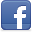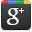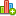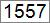Hello LearnerContributor
Stats
 Views : 19462 Comments : 0 Rating : 0
Actions

# Calculate Simple Interest using C#.NET

By Ashok Nalam on 27 Jun 2013 | Category: C# | Tagged: .NET number
The snippet covers program to Calculate Simple Interest using C#.NETAdd Comment   |Rate this resource

Simple Interest - Quickest method to calculate the interest on a loan. Simple Interest is calculated by multiplying the Interest Rate by the Principal by the Number of Periods.

Formula:

Simple Interest(SI) = P * I * N

P - Loan amount

I - Interest Rate

N - Duration of Loan Period

#### Program:

```namespace DotNetMirror
{
class SimpleInterestClass
{
static void Main()
{
double P, R, SI,N, finalAmount;
Console.Write("Enter Principal(Loan Amount) : ");
Console.Write("Enter Time Period(Years) : ");
Console.Write("Enter Rate Of Interest(%) : ");

SI = P * N * (R / 100); //formula
finalAmount = P + SI;

Console.WriteLine("Interest earned : {0}", SI);
Console.WriteLine("Final amount after interest : {0}", finalAmount);

}
}
}```

#### Output:

Enter Principal(Loan Amount) : 2000
Enter Time Period(Years) : 2
Enter Rate Of Interest(%) : 12
Interest earned : 480
Final amount after interest : 2480

Note: Simple Interest does not calculate based on compounding. Interest amount will be always on Principal, so Interest on Interest is not included. Simple Interest will be useful for Short Term periods.Add CommentRate this resource
 Name Email WebSite